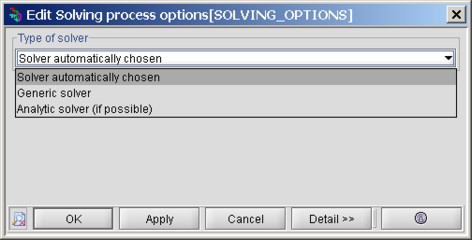# Choice of the solver

## Type of solver

There are two kinds of solver in Flux PEEC:

• the analytic solver which can only be used if all conductors and their profiles are parallel to the axis Ox, Oy or Oz of the global coordinate system. In fact, it computes the self and mutual inductances with analytical formulas of the PEEC method
• the generic solver which can be used for any computation (if there is no ground plane in the geometry) as it computes the inductive couplings with semi-analytical formulas based on the Gauss points approximation

## Choice of the solver

The user has three possible choices for the solver:

• solver automatically chosen by Flux PEEC (default option). In that case, Flux PEEC first analyzes the geometry of the project and if there is no ground plane, the generic solver is chosen. Otherwise the analytic solver is chosen
• generic solver
• analytic solver (if possible)

## Activation

The choice of the solver is accessible through this window: